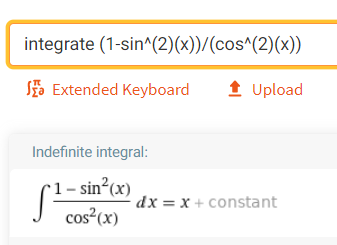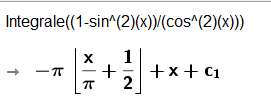# Integral problem

zhuzhaoheng shared this problem 2 years ago
Not a Problem

GeoGebra cannot integrate the function (1-sin^(2)(x))/(cos^(2)(x)) https://www.geogebra.org/cl...1

Thank you, looped to the Support Team@Mikeggb v5 CAS1

It's giving that answer because of the discontinuity. Try

`Assume(-pi/2<x<pi/2,Integral((1-sin^(2)(x))/(cos^(2)(x))))`1

or integral(simplify(f)) being f your function